### The Inverse Autocorrelation Function

The sample inverse autocorrelation function (SIACF) plays much the same role in ARIMA modeling as the sample partial autocorrelation function (SPACF), but it generally indicates subset and seasonal autoregressive models better than the SPACF.

Additionally, the SIACF can be useful for detecting over-differencing. If the data come from a nonstationary or nearly nonstationary model, the SIACF has the characteristics of a noninvertible moving-average. Likewise, if the data come from a model with a noninvertible moving average, then the SIACF has nonstationary characteristics and therefore decays slowly. In particular, if the data have been over-differenced, the SIACF looks like a SACF from a nonstationary process.

The inverse autocorrelation function is not often discussed in textbooks, so a brief description is given here. More complete discussions can be found in Cleveland (1972), Chatfield (1980), and Priestly (1981).

Let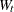be generated by the ARMA(p, q ) process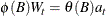where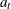is a white noise sequence. If(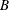) is invertible (that is, ifconsidered as a polynomial inhas no roots less than or equal to 1 in magnitude), then the model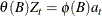is also a valid ARMA(q,p ) model. This model is sometimes referred to as the dual model. The autocorrelation function (ACF) of this dual model is called the inverse autocorrelation function (IACF) of the original model.

Notice that if the original model is a pure autoregressive model, then the IACF is an ACF that corresponds to a pure moving-average model. Thus, it cuts off sharply when the lag is greater than p; this behavior is similar to the behavior of the partial autocorrelation function (PACF).

The sample inverse autocorrelation function (SIACF) is estimated in the ARIMA procedure by the following steps. A high-order autoregressive model is fit to the data by means of the Yule-Walker equations. The order of the autoregressive model used to calculate the SIACF is the minimum of the NLAG= value and one-half the number of observations after differencing. The SIACF is then calculated as the autocorrelation function that corresponds to this autoregressive operator when treated as a moving-average operator. That is, the autoregressive coefficients are convolved with themselves and treated as autocovariances.

Under certain conditions, the sampling distribution of the SIACF can be approximated by the sampling distribution of the SACF of the dual model (Bhansali 1980). In the plots generated by ARIMA, the confidence limit marks (.) are located at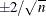. These limits bound an approximate 95% confidence interval for the hypothesis that the data are from a white noise process.